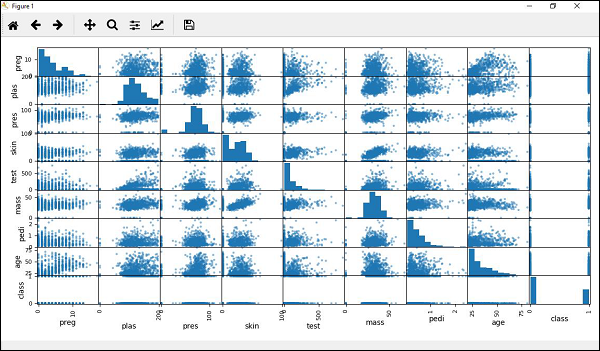# Machine Learning - Scatter Matrix Plot

Scatter plots shows how much one variable is affected by another or the relationship between them with the help of dots in two dimensions. Scatter plots are very much like line graphs in the concept that they use horizontal and vertical axes to plot data points.

## Example

In the following example, Python script will generate and plot Scatter matrix for the Pima Indian Diabetes dataset. It can be generated with the help of scatter_matrix() function on Pandas DataFrame and plotted with the help of pyplot.

from matplotlib import pyplot
from pandas.tools.plotting import scatter_matrix
path = r"C:\pima-indians-diabetes.csv"
names = ['preg', 'plas', 'pres', 'skin', 'test', 'mass', 'pedi', 'age', 'class']
data = read_csv(path, names = names)
scatter_matrix(data)
pyplot.show()machine_learning_with_python_understanding_data_with_visualization.htm